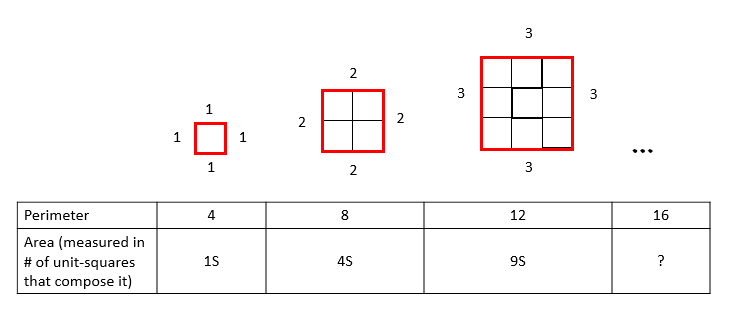Geometry

# Similar Polygons - Area and Perimeter Relations

The area of a hexagon is 6. What is the area of a similar hexagon whose side length is $5$ times as large?

Consider a $6 \times 10$ rectangle that has perimeter 32. If a similar rectangle has perimeter 16, what is its area?When the side-length of a square increases steadily, the perimeter and area increase as well.

If the side length increases in the sequence: 1, 2, 3, 4... then the perimeter increases in the sequence: 4, 8, 12, 16... and area, measured in unit-squares, increases in the sequence: 1, 4, 9, $\fbox{?}$

What number continues this pattern & is the area of a square with perimeter 16?

Two polygons are similar with side lengths in the ratio of $2 : 5$. What is the ratio of their areas?

When the side-lengths of a trapezoid increases steadily and proportionally, the perimeter and area increase as well.

If the side length of the top edge increases in the sequence: 1, 2, 3, 4... then the perimeter increases in the sequence: 5, 10, 15, 20... and area, measured in unit-trapezoids, increases in the sequence: 1, 4, 9, $\fbox{?}$

What number continues this pattern & is the area of a trapezoid (measured in unit-trapezoid) with perimeter 20?

×